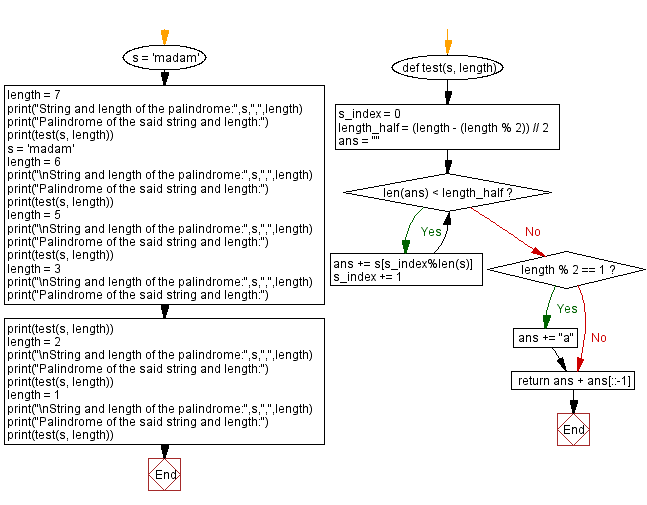﻿ Python: Find a palindrome of a given length containing a given string - w3resource# Python: Find a palindrome of a given length containing a given string

## Python Programming Puzzles: Exercise-95 with Solution

Write a Python program to find a palindrome of a given length containing a given string.

```Input:  madam , 7
Output:

Output:

Output:
maaaam

Output:
maam

Output:
mm

Output:
aa
```

Sample Solution:

Python Code:

``````#License: https://bit.ly/3oLErEI

def test(s, length):
s_index = 0
length_half = (length - (length % 2)) // 2
ans = ""
while len(ans) < length_half:
ans += s[s_index%len(s)]
s_index += 1
if length % 2 == 1:
ans += "a"
return ans + ans[::-1]

length = 7
print("String and length of the palindrome:",s,",",length)
print("Palindrome of the said string and length:")
print(test(s, length))
length = 6
print("\nString and length of the palindrome:",s,",",length)
print("Palindrome of the said string and length:")
print(test(s, length))
length = 5
print("\nString and length of the palindrome:",s,",",length)
print("Palindrome of the said string and length:")
print(test(s, length))
length = 3
print("\nString and length of the palindrome:",s,",",length)
print("Palindrome of the said string and length:")
print(test(s, length))
length = 2
print("\nString and length of the palindrome:",s,",",length)
print("Palindrome of the said string and length:")
print(test(s, length))
length = 1
print("\nString and length of the palindrome:",s,",",length)
print("Palindrome of the said string and length:")
print(test(s, length))
``````

Sample Output:

```String and length of the palindrome: madam , 7
Palindrome of the said string and length:

String and length of the palindrome: madam , 6
Palindrome of the said string and length:

String and length of the palindrome: madam , 5
Palindrome of the said string and length:
maaaam

String and length of the palindrome: madam , 3
Palindrome of the said string and length:
maam

String and length of the palindrome: madam , 2
Palindrome of the said string and length:
mm

String and length of the palindrome: madam , 1
Palindrome of the said string and length:
aa
```

Flowchart:## Visualize Python code execution:

The following tool visualize what the computer is doing step-by-step as it executes the said program:

Python Code Editor :

Have another way to solve this solution? Contribute your code (and comments) through Disqus.

What is the difficulty level of this exercise?

Test your Programming skills with w3resource's quiz.

﻿

## Python: Tips of the Day

Clamps num within the inclusive range specified by the boundary values x and y:

Example:

```def tips_clamp_num(num,x,y):
return max(min(num, max(x, y)), min(x, y))
print(tips_clamp_num(2, 4, 6))
print(tips_clamp_num(1, -1, -6))
```

Output:

```4
-1
```